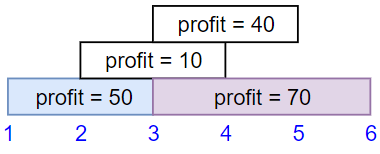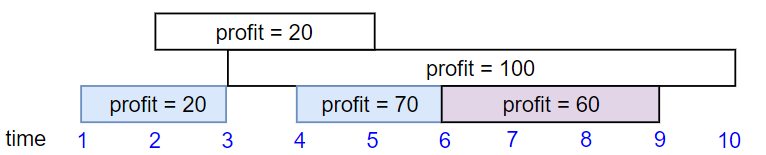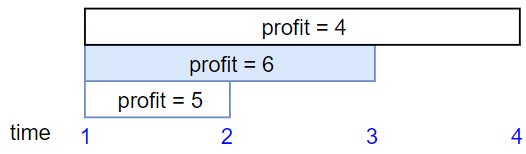Formatted question description: https://leetcode.ca/all/1235.html

# 1235. Maximum Profit in Job Scheduling (Hard)

We have n jobs, where every job is scheduled to be done from startTime[i] to endTime[i], obtaining a profit of profit[i].

You're given the startTime , endTime and profit arrays, you need to output the maximum profit you can take such that there are no 2 jobs in the subset with overlapping time range.

If you choose a job that ends at time X you will be able to start another job that starts at time X.

Example 1:Input: startTime = [1,2,3,3], endTime = [3,4,5,6], profit = [50,10,40,70]
Output: 120
Explanation: The subset chosen is the first and fourth job.
Time range [1-3]+[3-6] , we get profit of 120 = 50 + 70.


Example 2:Input: startTime = [1,2,3,4,6], endTime = [3,5,10,6,9], profit = [20,20,100,70,60]
Output: 150
Explanation: The subset chosen is the first, fourth and fifth job.
Profit obtained 150 = 20 + 70 + 60.


Example 3:Input: startTime = [1,1,1], endTime = [2,3,4], profit = [5,6,4]
Output: 6


Constraints:

• 1 <= startTime.length == endTime.length == profit.length <= 5 * 10^4
• 1 <= startTime[i] < endTime[i] <= 10^9
• 1 <= profit[i] <= 10^4

Related Topics: Binary Search, Dynamic Programming, Sort

// OJ: https://leetcode.com/problems/maximum-profit-in-job-scheduling/
// Time: O(NlogN)
// Space: O(N)
class Solution {
public:
int jobScheduling(vector<int>& startTime, vector<int>& endTime, vector<int>& profit) {
int N = startTime.size();
vector<vector<int>> v(N, vector<int>(3));
for (int i = 0; i < N; ++i) {
v[i] = startTime[i];
v[i] = endTime[i];
v[i] = profit[i];
}
sort(v.begin(), v.end()); // sort in asending order of start time.
vector<int> dp(N + 1);
for (int i = N - 1; i >= 0; --i) {
dp[i] = dp[i + 1]; // roll the max profit over
int e = v[i];
vector<int> c{ e, e, 0 }; // find the first job whose startTime is greater than or equal to the end time of the current job
int j = lower_bound(v.begin(), v.end(), c) - v.begin(); // upper_bound also works because it's impossible to be equal to the job e, e, 0.
dp[i] = max(dp[i], dp[j] + v[i]);
}
return dp;
}
};


Or

// OJ: https://leetcode.com/problems/maximum-profit-in-job-scheduling/
// Time: O(NlogN)
// Space: O(N)
// Ref: https://leetcode.com/problems/maximum-profit-in-job-scheduling/discuss/409188/C%2B%2B-with-picture
class Solution {
public:
int jobScheduling(vector<int>& startTime, vector<int>& endTime, vector<int>& profit) {
map<int, int> maxProfit; // start time to max profit
unordered_map<int, vector<pair<int, int>>> jobs; // start time to <endTime, profit>
for (int i = 0; i < startTime.size(); ++i) {
maxProfit[startTime[i]] = 0;
jobs[startTime[i]].emplace_back(endTime[i], profit[i]);
}
int ans = 0;
for (auto it = rbegin(maxProfit); it != rend(maxProfit); ++it) {
for (auto &job : jobs[it->first]) {
auto j = maxProfit.lower_bound(job.first); // find the first job whose start time is greater than or equal to the end time of this current job.
ans = max(ans, (j == end(maxProfit) ? 0 : j->second) + job.second);
}
it->second = ans;
}
return ans;
}
};


Using the ID array idx saves space compared to saving endTime and profit in another map as shown in the above solutions.

// OJ: https://leetcode.com/problems/maximum-profit-in-job-scheduling/
// Time: O(NlogN)
// Space: O(N)
// Ref: https://leetcode.com/problems/maximum-profit-in-job-scheduling/discuss/409188/C%2B%2B-with-picture
class Solution {
public:
int jobScheduling(vector<int>& startTime, vector<int>& endTime, vector<int>& profit) {
vector<int> idx(startTime.size());
iota(begin(idx), end(idx), 0);
sort(begin(idx), end(idx), [&](int i, int j) { return startTime[i] > startTime[j]; }); // sort the job ids in descending order of start time.
map<int, int> maxProfit; // start time to max profit
int ans = 0;
for (int i = 0; i < idx.size(); ++i) {
auto j = maxProfit.lower_bound(endTime[idx[i]]); // find the first job whose start time is greater than or equal to the end time of the current job.
ans = max(ans, (j == end(maxProfit) ? 0 : j->second) + profit[idx[i]]);
maxProfit[startTime[idx[i]]] = ans;
}
return ans;
}
};


## Solution 1. DP + Binary Search

For a job with startTime s, endTime e, and profit p, we need look at all the jobs ends at and before s.

Let dp[s] be the maximum profit we can get from the beginning to time s, then we can try to update dp[e] with dp[s] + p.

But dp[s] is not necessarily set because there might be no jobs ending at s, so we need to find the maximum time t <= s, and use dp[t].

To achieve this, we can keep the dp values sorted in ascending order of the key, i.e. time.

To ensure we’ve visited all the jobs ends before startTime s, we sort the jobs in ascending order of endTime.

// OJ: https://leetcode.com/problems/maximum-profit-in-job-scheduling/
// Time: O(NlogN)
// Space: O(N)
class Solution {
public:
int jobScheduling(vector<int>& startTime, vector<int>& endTime, vector<int>& profit) {
vector<array<int, 3>> jobs;
for (int i = 0; i < startTime.size(); ++i) jobs.push_back({ endTime[i], startTime[i], profit[i] });
sort(begin(jobs), end(jobs));
map<int, int> dp{ {0, 0} }; // endTime to max profit
int ans = 0;
for (auto &[e, s, p] : jobs) {
dp[e] = max(ans, p + prev(dp.upper_bound(s))->second);
ans = max(ans, dp[e]);
}
return ans;
}
};


Or

// OJ: https://leetcode.com/problems/maximum-profit-in-job-scheduling/
// Time: O(NlogN)
// Space: O(N)
class Solution {
public:
int jobScheduling(vector<int>& startTime, vector<int>& endTime, vector<int>& profit) {
int ans = 0, N = startTime.size();
vector<array<int, 3>> jobs(N + 1);
for (int i = 0; i < N; ++i) jobs[i + 1] = { endTime[i], startTime[i], profit[i] };
sort(begin(jobs), end(jobs));
vector<int> dp(N + 1);
for (int i = 1; i <= N; ++i) {
auto [e, s, p] = jobs[i];
int j = prev(upper_bound(begin(jobs), end(jobs), array<int, 3>{s, s, 0})) - begin(jobs);
dp[i] = max(ans, p + dp[j]);
ans = max(ans, dp[i]);
}
return ans;
}
};


Another way to get the upper_bound is as follows

upper_bound(begin(jobs), end(jobs), s, [](int a, auto &b) { return a < b; })


The other way around also works, i.e. sorting the jobs based on the start times and get the dp values in descending order of time.

// OJ: https://leetcode.com/problems/maximum-profit-in-job-scheduling/
// Time: O(NlogN)
// Space: O(N)
class Solution {
public:
int jobScheduling(vector<int>& startTime, vector<int>& endTime, vector<int>& profit) {
vector<array<int, 3>> jobs;
for (int i = 0; i < startTime.size(); ++i) jobs.push_back({ startTime[i], endTime[i], profit[i] });
sort(begin(jobs), end(jobs), greater<>());
map<int, int> dp{ {INT_MAX, 0} }; // startTime to max profit
int ans = 0;
for (auto &[s, e, p] : jobs) {
dp[s] = max(ans, p + dp.lower_bound(e)->second);
ans = max(ans, dp[s]);
}
return ans;
}
};


Or

// OJ: https://leetcode.com/problems/maximum-profit-in-job-scheduling/
// Time: O(NlogN)
// Space: O(N)
class Solution {
public:
int jobScheduling(vector<int>& startTime, vector<int>& endTime, vector<int>& profit) {
int ans = 0, N = startTime.size();
vector<array<int, 3>> jobs(N + 1);
jobs[N] = { INT_MAX, INT_MAX, 0 };
for (int i = 0; i < N; ++i) jobs[i] = { startTime[i], endTime[i], profit[i] };
sort(begin(jobs), end(jobs));
vector<int> dp(N + 1);
for (int i = N - 1; i >= 0; --i) {
auto [s, e, p] = jobs[i];
int j = lower_bound(begin(jobs), end(jobs), array<int, 3>{e, e, 0}) - begin(jobs);
dp[i] = max(ans, p + dp[j]);
ans = max(ans, dp[i]);
}
return ans;
}
};


Java

• class Solution {
public int jobScheduling(int[] startTime, int[] endTime, int[] profit) {
int length = startTime.length;
int[][] jobs = new int[length];
for (int i = 0; i < length; i++) {
jobs[i] = startTime[i];
jobs[i] = endTime[i];
jobs[i] = profit[i];
}
Arrays.sort(jobs, new Comparator<int[]>() {
public int compare(int[] job1, int[] job2) {
return job1 - job2;
}
});
int[] prevJobs = new int[length];
for (int i = 0; i < length; i++)
prevJobs[i] = binarySearch(jobs, jobs[i]);
int[][] dp = new int[length];
dp = 0;
dp = jobs;
for (int i = 1; i < length; i++) {
dp[i] = Math.max(dp[i - 1], dp[i - 1]);
int prevJob = prevJobs[i];
if (prevJob >= 0)
dp[i] = Math.max(dp[prevJob], dp[prevJob]) + jobs[i];
else
dp[i] = jobs[i];
}
return Math.max(dp[length - 1], dp[length - 1]);
}

public int binarySearch(int[][] jobs, int maxEndTime) {
int low = 0, high = jobs.length - 1;
while (low <= high) {
int mid = (high - low + 1) / 2 + low;
int[] job = jobs[mid];
if (job > maxEndTime)
high = mid - 1;
else {
if (low == high)
break;
else
low = mid;
}
}
return high;
}
}

• // OJ: https://leetcode.com/problems/maximum-profit-in-job-scheduling/
// Time: O(NlogN)
// Space: O(N)
class Solution {
public:
int jobScheduling(vector<int>& startTime, vector<int>& endTime, vector<int>& profit) {
vector<array<int, 3>> jobs;
for (int i = 0; i < startTime.size(); ++i) jobs.push_back({ startTime[i], endTime[i], profit[i] });
sort(begin(jobs), end(jobs), greater<>()); // sort jobs in descending order of start time
map<int, int> dp{ {INT_MAX, 0} }; // startTime to max profit
int ans = 0;
for (auto &[s, e, p] : jobs) {
dp[s] = max(ans, p + dp.lower_bound(e)->second);
ans = max(ans, dp[s]);
}
return ans;
}
};

• print("Todo!")